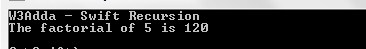# Swift Recursion

In this tutorial you will learn about the Swift Recursion and its application with practical example.

## Swift Recursion

Recursion is the process where a function calls itself, and the function which calls itself is know as recursive function.

## Characteristics of a recursive function

• A recursive function is a function which calls itself.
• The speed of a recursive program is slower because of stack overheads.
• A recursive function must have terminating conditions, and recursive expressions.

• It requires few variables which make program clean.
• It shorten the complex and nested code.

• It is hard to debug recursive function.
• It is tough to understand the logic of a recursive function.

To understand how recursion works lets have one of the popular example of recursion. In this example we will calculate the factorial of n numbers. The factorial of n numbers is expressed as a series of repetitive multiplication as shown below:

Example:

Example:-

Output:-In this tutorial we have learn about the Swift Recursion and its application with practical example. I hope you will like this tutorial.